Documentation

JavaFX: Working with JavaFX UI Components

# 33 Area Chart

This chapter describes the area chart, yet another type of a two-axis chart.

Similar to line charts, it presents data as a series of points connected by straight lines. However, the area between the axis and the line is painted with color. Each series of data is painted with a different color. Figure 33-1 shows an area chart with two series of data.

Figure 33-1 Typical Area Chart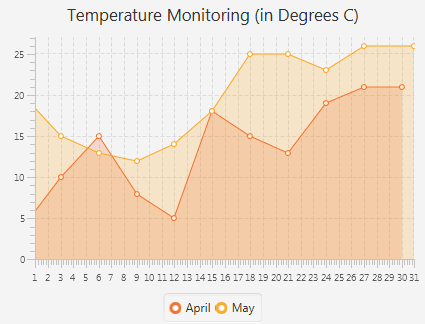Description of "Figure 33-1 Typical Area Chart"

## Creating an Area Chart

To create a simple area chart in your application, at minimum, you must define two axes, create the `AreaChart` object by instantiating the `AreaChart` class, create one or more series of data by using the `XYChart.Series` class, and assign the data to the chart.

When instantiating the `AreaChart` class, you can specify the observable list with a series of data within a constructor, or add the series later by calling the `getData` and `addAll` methods on the `AreaChart` object.

Example 33-1 creates an area chart to illustrate temperature monitoring data. The example uses two series of data collected for the periods of April and May.

Example 33-1 Creating an Area Chart

```import javafx.application.Application;
import javafx.scene.Scene;
import javafx.scene.chart.AreaChart;
import javafx.scene.chart.NumberAxis;
import javafx.scene.chart.XYChart;
import javafx.stage.Stage;

public class AreaChartSample extends Application {

@Override public void start(Stage stage) {
stage.setTitle("Area Chart Sample");
final NumberAxis xAxis = new NumberAxis(1, 31, 1);
final NumberAxis yAxis = new NumberAxis();
final AreaChart<Number,Number> ac =
new AreaChart<>(xAxis,yAxis);
ac.setTitle("Temperature Monitoring (in Degrees C)");

XYChart.Series seriesApril= new XYChart.Series();
seriesApril.setName("April");
seriesApril.getData().add(new XYChart.Data(1, 4));
seriesApril.getData().add(new XYChart.Data(3, 10));
seriesApril.getData().add(new XYChart.Data(6, 15));
seriesApril.getData().add(new XYChart.Data(9, 8));
seriesApril.getData().add(new XYChart.Data(12, 5));
seriesApril.getData().add(new XYChart.Data(15, 18));
seriesApril.getData().add(new XYChart.Data(18, 15));
seriesApril.getData().add(new XYChart.Data(21, 13));
seriesApril.getData().add(new XYChart.Data(24, 19));
seriesApril.getData().add(new XYChart.Data(27, 21));
seriesApril.getData().add(new XYChart.Data(30, 21));

XYChart.Series seriesMay = new XYChart.Series();
seriesMay.setName("May");
seriesMay.getData().add(new XYChart.Data(1, 20));
seriesMay.getData().add(new XYChart.Data(3, 15));
seriesMay.getData().add(new XYChart.Data(6, 13));
seriesMay.getData().add(new XYChart.Data(9, 12));
seriesMay.getData().add(new XYChart.Data(12, 14));
seriesMay.getData().add(new XYChart.Data(15, 18));
seriesMay.getData().add(new XYChart.Data(18, 25));
seriesMay.getData().add(new XYChart.Data(21, 25));
seriesMay.getData().add(new XYChart.Data(24, 23));
seriesMay.getData().add(new XYChart.Data(27, 26));
seriesMay.getData().add(new XYChart.Data(31, 26));

Scene scene  = new Scene(ac,800,600);
ac.getData().addAll(seriesApril, seriesMay);
stage.setScene(scene);
stage.show();
}

public static void main(String[] args) {
launch(args);
}
}
```

This example creates two `NumberAxis` objects to present numerical data on horizontal and vertical axes. Values rendered on the horizontal axis (X) are retrieved from the first parameter of the `XYChart.Data` objects, whereas the second parameter provides data for the vertical axis (Y).

The series of data is assigned to the chart by using the `getData` and `addAll` methods. Because the `seriesMay` data is added last, the corresponding green area overlays the yellow area that shows April data.

The result of compiling and running the application, is shown in Figure 33-2.

Figure 33-2 Area Chart with Two Series of Data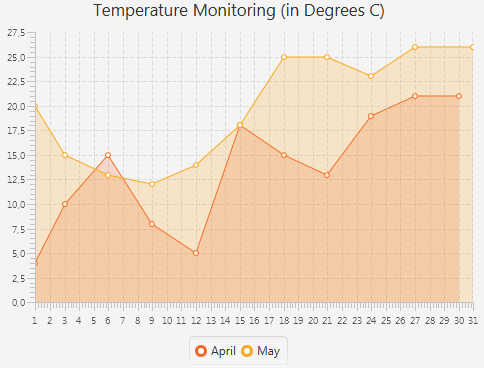Description of "Figure 33-2 Area Chart with Two Series of Data"

## Creating a Stacked Area Chart

You can represent data in the area chart by using the `StackedAreaChart` class. This class builds areas that are stacked so that each series adjoins but does not overlap the preceding series. Example 33-2 implements this task.

Example 33-2 Creating a Stacked Area Chart

```import javafx.application.Application;
import javafx.scene.Scene;
import javafx.scene.chart.NumberAxis;
import javafx.scene.chart.StackedAreaChart;
import javafx.scene.chart.XYChart;
import javafx.stage.Stage;

public class StackedAreaChartSample extends Application {
final NumberAxis xAxis = new NumberAxis(1, 31, 1);
final NumberAxis yAxis = new NumberAxis();
final StackedAreaChart<Number, Number> sac =
new StackedAreaChart<>(xAxis, yAxis);

@Override
public void start(Stage stage) {
stage.setTitle("Area Chart Sample");
sac.setTitle("Temperature Monitoring (in Degrees C)");
XYChart.Series<Number, Number> seriesApril =
new XYChart.Series<>();
seriesApril.setName("April");
seriesApril.getData().add(new XYChart.Data(1, 4));
seriesApril.getData().add(new XYChart.Data(3, 10));
seriesApril.getData().add(new XYChart.Data(6, 15));
seriesApril.getData().add(new XYChart.Data(9, 8));
seriesApril.getData().add(new XYChart.Data(12, 5));
seriesApril.getData().add(new XYChart.Data(15, 18));
seriesApril.getData().add(new XYChart.Data(18, 15));
seriesApril.getData().add(new XYChart.Data(21, 13));
seriesApril.getData().add(new XYChart.Data(24, 19));
seriesApril.getData().add(new XYChart.Data(27, 21));
seriesApril.getData().add(new XYChart.Data(30, 21));
XYChart.Series<Number, Number> seriesMay =
new XYChart.Series<>();
seriesMay.setName("May");
seriesMay.getData().add(new XYChart.Data(1, 20));
seriesMay.getData().add(new XYChart.Data(3, 15));
seriesMay.getData().add(new XYChart.Data(6, 13));
seriesMay.getData().add(new XYChart.Data(9, 12));
seriesMay.getData().add(new XYChart.Data(12, 14));
seriesMay.getData().add(new XYChart.Data(15, 18));
seriesMay.getData().add(new XYChart.Data(18, 25));
seriesMay.getData().add(new XYChart.Data(21, 25));
seriesMay.getData().add(new XYChart.Data(24, 23));
seriesMay.getData().add(new XYChart.Data(27, 26));
seriesMay.getData().add(new XYChart.Data(31, 26));
Scene scene = new Scene(sac, 800, 600);
sac.getData().addAll(seriesApril, seriesMay);
stage.setScene(scene);
stage.show();
}

public static void main(String[] args) {
launch(args);
}
}
```

When you compile and run this application, it creates the chart shown in Figure 33-3.

Figure 33-3 Stacked Area Chart with Two Areas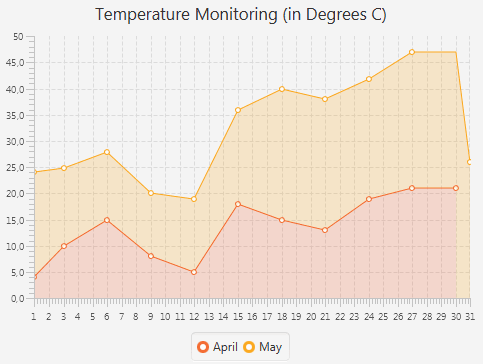Description of "Figure 33-3 Stacked Area Chart with Two Areas"

Compare the data shown in Figure 33-3 with the same data in Figure 33-2. The areas in the stacked area chart show cumulative values on the vertical axis at any given point along the horizontal axis. For example, the value on the vertical axis shown for May 15th in the stacked area chart is 36, which does not correspond to the actual temperature that day. This value represents the cumulative result for temperatures on April 15th and May 15th.

When you develop area charts in your JavaFX applications, remember that data on the vertical axes is interpreted according to the type of area charts (`AreaChart` or `StackedAreaChart`). Choose the data representation best suited for the task of the application.

## Setting Axis and Tick Properties

The output of the Temperature Monitoring application in Figure 33-2 and Figure 33-3 presents the numerical values on the axes in the default double format., rather than in a user-friendly manner. For example, the month days should be integers and in the range of 1 to 31, instead of float numbers.

The JavaFX SDK API provides several methods to adjust the appearance of values rendered on chart axes. Figure 33-4 shows the main elements of the chart axis, including tick marks and tick labels that indicate numeric values of the range.

Figure 33-4 Elements of an Axis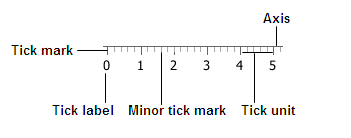Description of "Figure 33-4 Elements of an Axis"

You can specify the minimum and maximum values in the numerical range by using a constructor of the `NumberAxis` class or the corresponding methods, as shown in Example 33-3.

Example 33-3 Specifying a Data Range for the Horizontal Axis

```//Using the NumberAxis constructor
final NumberAxis xAxis = new NumberAxis(1, 31, 1);
//Using the corresponding methods
xAxis.setLowerBound(1);
xAxis.setUpperBound(31);
xAxis.setTickUnit(1);
```

When using the three-parameter constructor of the `NumberAxis` class, remember that the first parameter defines the minimum value in the range, the second parameter is the maximum value in the range, and the third parameter defines the tick unit, a value between two tick marks on the axis.

Additionally, if you want to prohibit showing minor ticks on the horizontal axis, then specify 0 for the `minorTickCount` property, as shown in Example 33-4.

Example 33-4 Setting Zero Value for Minor Tick Count

```xAxis.setMinorTickCount(0);
```

This property defines the number of minor ticks to be displayed between each major tick mark. By setting its value to 0, you disable the minor ticks for the horizontal axis.

When you add the code lines from Example 33-3 and Example 33-4 to the Temperature Monitoring application, the horizontal axis changes as shown in Figure 33-5.

Figure 33-5 Setting the Horizontal Axis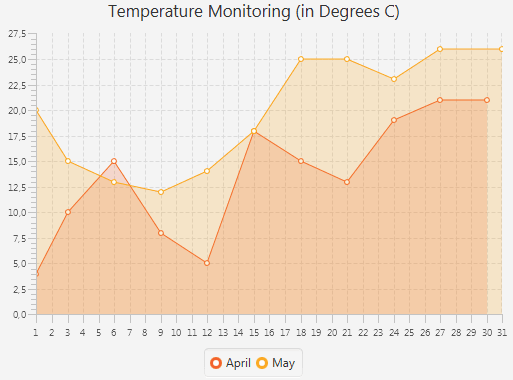Description of "Figure 33-5 Setting the Horizontal Axis"

If your application requires no tick labels to be shown, use the `setTickLabelsVisible` method with the `false` value. Similarly, use `setTickMarkVisible` method with the `false` value if you do not want tick marks to be visible.

Use the code line shown in Example 33-5 to adjust the range of values for the vertical axis.

Example 33-5 Specifying a Data Range for the Vertical Axis

```final NumberAxis yAxis = new NumberAxis(0, 27, 5);
```

You can also adjust tick marks so that minor and major tick marks have equal length. Use the `tickLength` and `minorTickLength` properties as shown in Example 33-6.

Example 33-6 Adjusting the Length of Major and Minor Tick Marks

```yAxis.setMinorTickLength(yAxis.getTickLength());
```

When you add code lines from Example 33-5 and Example 33-6 to the Temperature Monitoring application, the vertical axes changes as shown in Figure 33-6.

Figure 33-6 Setting the Vertical Axis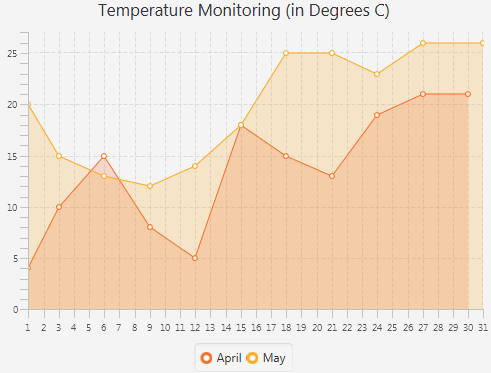Description of "Figure 33-6 Setting the Vertical Axis"

## Adding Negative Values

Because the vertical axis in the Temperature Monitoring application is created by using the `NumberAxis` class, you can specify negative values for the area chart data.

Create one more series of data as shown in Example 33-7.

Example 33-7 Adding a Series of Data with Negative Values

```import javafx.application.Application;
import javafx.scene.Scene;
import javafx.scene.chart.AreaChart;
import javafx.scene.chart.NumberAxis;
import javafx.scene.chart.XYChart;
import javafx.stage.Stage;

public class AreaChartSample extends Application {

@Override public void start(Stage stage) {
stage.setTitle("Area Chart Sample");
final NumberAxis xAxis = new NumberAxis(1, 31, 1);
xAxis.setMinorTickCount(0);
final NumberAxis yAxis = new NumberAxis(-5, 27, 5);
yAxis.setMinorTickLength(yAxis.getTickLength());
yAxis.setForceZeroInRange(false);

final AreaChart<Number,Number> ac =
new AreaChart<Number,Number>(xAxis,yAxis);
ac.setTitle("Temperature Monitoring (in Degrees C)");

XYChart.Series seriesApril= new XYChart.Series();
seriesApril.setName("April");
seriesApril.getData().add(new XYChart.Data(0, 4));
seriesApril.getData().add(new XYChart.Data(3, 10));
seriesApril.getData().add(new XYChart.Data(6, 15));
seriesApril.getData().add(new XYChart.Data(9, 8));
seriesApril.getData().add(new XYChart.Data(12, 5));
seriesApril.getData().add(new XYChart.Data(15, 18));
seriesApril.getData().add(new XYChart.Data(18, 15));
seriesApril.getData().add(new XYChart.Data(21, 13));
seriesApril.getData().add(new XYChart.Data(24, 19));
seriesApril.getData().add(new XYChart.Data(27, 21));
seriesApril.getData().add(new XYChart.Data(30, 21));

XYChart.Series seriesMay = new XYChart.Series();
seriesMay.setName("May");
seriesMay.getData().add(new XYChart.Data(0, 20));
seriesMay.getData().add(new XYChart.Data(3, 15));
seriesMay.getData().add(new XYChart.Data(6, 13));
seriesMay.getData().add(new XYChart.Data(9, 12));
seriesMay.getData().add(new XYChart.Data(12, 14));
seriesMay.getData().add(new XYChart.Data(15, 18));
seriesMay.getData().add(new XYChart.Data(18, 25));
seriesMay.getData().add(new XYChart.Data(21, 25));
seriesMay.getData().add(new XYChart.Data(24, 23));
seriesMay.getData().add(new XYChart.Data(27, 26));
seriesMay.getData().add(new XYChart.Data(31, 26));

XYChart.Series seriesMarch = new XYChart.Series();
seriesMarch.setName("March");
seriesMarch.getData().add(new XYChart.Data(0, -2));
seriesMarch.getData().add(new XYChart.Data(3, -4));
seriesMarch.getData().add(new XYChart.Data(6, 0));
seriesMarch.getData().add(new XYChart.Data(9, 5));
seriesMarch.getData().add(new XYChart.Data(12, -4));
seriesMarch.getData().add(new XYChart.Data(15, 6));
seriesMarch.getData().add(new XYChart.Data(18, 8));
seriesMarch.getData().add(new XYChart.Data(21, 14));
seriesMarch.getData().add(new XYChart.Data(24, 4));
seriesMarch.getData().add(new XYChart.Data(27, 6));
seriesMarch.getData().add(new XYChart.Data(31, 6));

Scene scene  = new Scene(ac,800,600);
ac.getData().addAll(seriesMarch, seriesApril, seriesMay);
stage.setScene(scene);
stage.show();
}

public static void main(String[] args) {
launch(args);
}
}
```

Figure 33-7 demonstrates the Temperature Monitoring application modified to display the weather data for three months: March, April, and May.

Figure 33-7 Adding Negative Data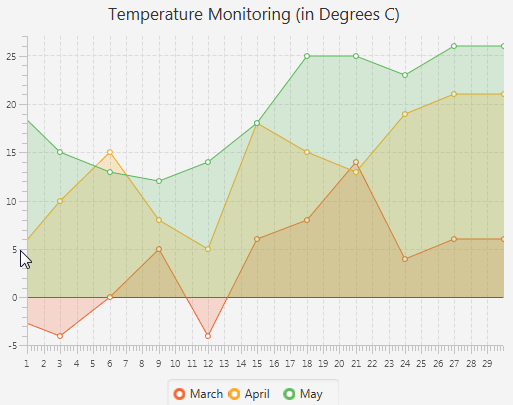Description of "Figure 33-7 Adding Negative Data"

## Styling Area Charts

The color for each month in Example 33-7 is defined by the order of the corresponding data series as declared in the `addAll` method. That is why the March area in Figure 33-7 is painted yellow. You can set the color for `AreaChart` objects through CSS.

Create the `Chart.css` file and save it in the same directory as the main class of the `AreaChartSample` application. Add the lines shown in Example 33-8 to the `Chart.css` file.

Example 33-8 CSS Styles for an Area Chart

```.default-color0.chart-area-symbol { -fx-background-color: #e9967a, #ffa07a; }
.default-color1.chart-area-symbol { -fx-background-color: #f0e68c, #fffacd; }
.default-color2.chart-area-symbol { -fx-background-color: #dda0dd, #d8bfd855; }

.default-color0.chart-series-area-line { -fx-stroke: #e9967a; }
.default-color1.chart-series-area-line { -fx-stroke: #f0e68c; }
.default-color2.chart-series-area-line { -fx-stroke: #dda0dd; }

.default-color0.chart-series-area-fill { -fx-fill: #ffa07a55; }
.default-color1.chart-series-area-fill { -fx-fill: #fffacd55; }
.default-color2.chart-series-area-fill { -fx-fill: #d8bfd855; }
```

The `chart-area-symbol` CSS class defines parameters of the symbol in the chart legend for a particular data series. Example 33-8 sets the inner and outer colors for the circles in the chart legend.

The `chart-series-area-line` CSS class sets parameters for the area chart lines. In this example, the color of the line stroke. The `chart-series-area-fill` CSS class defines the color and the opacity level of the areas.

These styles are applied to the `AreaChartSample` application by using the `getStylesheets()` method of the `Scene` class, as shown Example 33-9.

Example 33-9 Applying CSS Styles to the Scene

```scene.getStylesheets().add("areachartsample/Chart.css");
```

Compiling and running this application produces the modified appearance of the area chart shown in Figure 33-8.

Figure 33-8 Styled Area Chart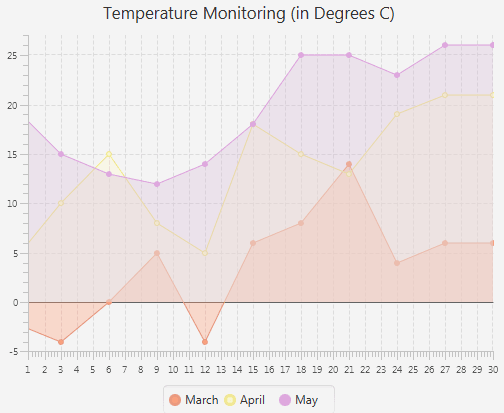Description of "Figure 33-8 Styled Area Chart"

You can learn more about using CSS styles in JavaFX applications from Styling Charts with CSS.

Related API Documentation

## JavaFX: Working with JavaFX UI Components

Expand | Collapse